## Friday, July 5, 2019

### Tips for Training Likelihood Models

This is a tutorial on common practices in training generative models that optimize likelihood directly, such as autoregressive models and normalizing flows. Deep generative modeling is a fast-moving field, so I hope for this to be a newcomer-friendly introduction to the basic evaluation terminology used consistently across research papers, especially when it comes to modeling more complicated distributions like RGB images. This is a more in-depth version of the tutorial lecture I gave on normalizing flows at ICML.

This tutorial discusses the most mathematically straightforward of generative models (tractable density estimation models), and cover some important design considerations when choosing how to model image pixels. By the end of this post, you will know how to quantitatively compare likelihood models, even ones that differ drastically in architecture and the way pixels are modeled.

### Divergence Minimization: A General Framework for Generative Modeling

The goal of generative modeling (all of statistical machine learning, really) is to take data sampled from some (possibly conditional) probability distribution $p(x)$ and learn a model $p_\theta(x)$ that approximates $p(x)$. Modeling allows us to extrapolate insight beyond the raw data we are given. Here are some versatile things you can do with generative models:
• Draw new samples from $p(x)$
• Learn hierarchical latent variables $z$ that explain the observations $x$
• You can intervene on latent variables to examine the interventionist distributions $p_\theta(x|do(z))$ Note that this will only work properly if your conditional distribution models the correct causal relationship $z \to x$ and we assume ignorability.
• Interrogate the likelihood of a new data point $x^\prime$ under our model distribution to detect anomalies
Modeling conditional distributions has an even broader set of direct applications, since we can interpret classification and regression problems as learning generative models:
• Machine Translation $p(\text{translated english sentence}|\text{french sentence})$
• Captioning $p(\text{caption}|\text{image})$
• Regression objectives like minimizing mean-squared error $\min \frac{1}{2}(x-\mu)^2$ are mathematically equivalent to maximum log-likelihood estimation of a Gaussian with diagonal covariance: $\max -\frac{1}{2} (x-\mu)^2$.

In order to get $p_\theta(x)$ to match $p(x)$, we first have to come up with the notion of a distance between the two distributions. In statistics, it is more common to devise a weaker notion of “distance” called a divergence measure, which unlike a metric distance, is not symmetric ($D(p, q) \neq D(q, p)$). Once we have a formal divergence measure between distributions can we attempt to minimize it via optimization.

There are many, many divergences $D(p_\theta || p)$ that we can formulate, and these are often chosen to suit the generative modeling algorithm. Here are just a few:
• Maximum Mean Discrepancy (MMD)
• Jensen-Shannon Divergence (JSD)
• Kullback-Leibler divergence (KLD)
• Reverse KLD
• Kernelized Stein discrepancy (KSD)
• Bregman Divergence
• Hyvärinen score
• Chi-Squared Divergence
• Alpha Divergence
Divergences between two distributions, unlike metrics, need not be symmetric. In the limit of infinite data and compute, all these divergences arrive at the same answer, that is, $D(p_\theta || p) = 0$ iff $p_\theta \equiv p$. Note that these divergences are distinct from perceptual evaluation metrics like Inception Score or Fréchet Inception Distance, which do not guarantee convergence to the same result in the high-data limit (but are useful metrics if you care about visual quality of images).

However, most experiments see a finite amount of data and compute, so the choice of metric matters and can actually change the qualitative behavior of what generative distribution $p_\theta(x)$ ends up being learned. For example, if the target density is $p$ is multi-modal and the model distribution $q$ is not expressive enough, minimizing forward KL $D_{KL}(p||q)$ will learn mode-covering behavior while minimizing reverse KL $D_{KL}(q||p)$ results in mode-dropping behavior. See this blog post for an explanation why.

Thinking about generative modeling in the framework of divergence minimization is useful because it allows us to map what we properties want from our generative models to our choice of divergence objective in a principled way. It may be an implicit density model (GANs) where sampling is tractable but log-probabilities are not available, or a energy-based model where sampling is not available but (unnormalized) log-probabilities are tractable.

This blog post will cover models trained and evaluated using the most straightforward metric: the Kullback-Leibler Divergence. These models include Autoregressive Models, Normalizing Flows, and Variational Autoencoders (approximately). Optimizing KLD is equivalent to optimizing log-probability, and we'll derive why in the next section!

### Average Log-Probability and Compression

We want to model $p(x)$, the probability distribution for some data-generating stochastic process. We typically assume that sampling from a sufficiently large dataset is approximately the same thing as sampling from the true data-generating process. For instance, sampling an image from the MNIST dataset is equivalent to drawing a sample from the true handwriting process that created the MNIST dataset.

Given a test set of images $x^1,...,x^N$ sampled i.i.d from $p(x)$, and a likelihood model $p_\theta$ parameterized by $\theta$, we want to maximize the following objective:

$$\mathcal{L}(\theta) = \frac{1}{N}\sum_{i=1}^{N}\log p_\theta(x^i) \sim \int p(x) \log p_\theta(x) dx = -H(p, p_\theta)$$

The average log-probability is a Monte Carlo estimator of the negative cross entropy between the true likelihood $p$ and model likelihood $p_\theta$, because we are not able to actually enumerate over all $x^i$. In plain language, this translates to "maximize average likelihood of data", or equivalently, "minimize negative cross-entropy between true distribution and model distribution".

With a little algebra, the negative cross-entropy can be re-written in terms of KL divergence (relative entropy) and absolute entropy of $p$:

$$-H(p, p_\theta) = -KL(p, p_\theta) - H(p)$$

Shannon’s Source Coding Theorem (1948) tells us that entropy $H(p)$ is the lower bound on average code length for any code you can construct to communicate samples from $p(x)$ losslessly. More entropy means more "randomness", which cannot be compressed. In particular, when we use the natural logarithm $\log_e$ to compute entropy, it takes on the "natural units of information", or nats. When computing entropy in $\log_2$, the resulting units are the familiar "bit". The $H(p)$ term is independent of $\theta$, so maximizing $\mathcal{L}(\theta)$ is really just equivalent to minimizing $KL(p, p_\theta)$. That is why maximum likelihood is also known as minimizing KL divergence.

The KL divergence $KL(p, p_\theta)$, or relative entropy, is the number of "extra nats" you would need to encode data from $p(x)$ using an entropy coding scheme based on $p_\theta(x)$. Therefore, our Monte Carlo estimator $\mathcal{L}(\theta)$ of negative cross entropy is also expressed in nats.

Putting the two together, the cross entropy is nothing more than the average code length required to communicate samples from $p$, using a codebook based on $p_\theta$. We pay a "base fee" of $H(p)$ nats no matter what (the optimal code), and we pay an additional "fine" of $KL(p, p_\theta)$ nats for any deviations of $p_\theta$ from $p$.

We can compare cross entropies of two different models in a very interpretable way: suppose model $\theta_1$ has average likelihood $\mathcal{L}(\theta_1)$ and model $\theta_2$ has average likelihood $\mathcal{L}(\theta_2)$. Subtracting $\mathcal{L}(\theta_1) - \mathcal{L}(\theta_2)$ causes the entropy terms $H(p)$ to cancel out, resulting in $KL(p, p_{\theta1})-KL(p, p_{\theta_2})$. This quantity is the "reduction of penalty nats you need to pay when switching from code $p_{\theta_1}$ to code $p_{\theta_2}$".

Expressivity, optimization, and generalization are three important properties of a good generative model, and likelihoods offer an interpretable metric with which to debug these properties in our models. If a generative model cannot memorize the training set, it suggests there are difficulties with optimization (getting stuck) or expressivity (underfitting).

The Cifar10 image dataset has 50000 training samples, so we know that a model that memorizes the data perfectly will assign a probability mass of exactly 1/50000 to each image in the training dataset, thereby achieving a negative cross entropy of $log_2(\frac{1}{50000})$, or 15.6 bits per image (this is independent of how many pixels there are per image!). Of course, we usually don’t want our generative models to overfit to such extremes, but it’s useful to keep this upper bound in mind as a sanity check when debugging your generative model.

Comparing the difference between training and test likelihoods can tell us if the networks are memorizing the training set or learning something that generalizes to the test set, or whether there are semantically meaningful modes in the data that the model fails to capture.

### Which Distribution Should You Use For Modeling Image Pixels?

There are plenty ways to parameterize an image. For instance, you can represent an image via a 3D scene that is projected (rendered) into 2D. Or you can parameterize images as vector representations of sketches (like SVG graphics), or Laplacian Pyramids, or even motor torques for a robotic arm that subsequently paint a picture. However, for simplicity, researchers typically models image likelihoods as the joint distribution over RGB pixels - it is a general-purpose digital format that has proven effective for capturing natural data in the visible electromagnetic spectrum.

Each RGB pixel is encoded by a uint8 integer, which can take on 256 possible values. Thus, an image with 3072 pixels and 256 possible values per pixel can take on $256^{3072}$ possible values. There are a finite number of images, which means we could technically represent images using a single $256^{3072}$-sided die. But this number is too large to be represented in memory! Even modeling 3 uint8-encoded pixels jointly as a Categorical results in $256^3=16777216$ possible categories, which is unwieldy even for modern computers. To make things computationally tractable, we must "factorize" the likelihood for the whole image into a combination of conditionally independent pixel-wise distributions. One easy factorization is to make each pixel likelihood independent of one another:

$$p(x_1, ..., x_N) = p(x_1)p(x_2)...p(x_N)$$

This is also known as a mean-field decoders (see this comment for where the name “mean-field” comes from). Each pixel-wise distribution has its own tractable density or mass function.

Another choice is to make the pixel likelihood autoregressive, where each conditional distribution has its own tractable density or mass function.

$$p(x_1, ..., x_N) = p(x_1)p(x_2|x_1)...p(x_N|x_1,...,x_{N-1})$$

We still have to figure out how to model each conditional distribution though. Here are some common choices along with an example paper that used them:
1. Bernoulli probabilities over each channel (DRAW)
2. 256-way Categorical distribution over each channel (PixelRNNImage Transformer)
3. Continuous density on de-quantized data (Real-NVP)
4. Discretized logistic mixture (PixelCNN++, Image Transformer)

#### Pixel Values as Bernoulli Emission Probabilities

• Those datasets can be stored completely in computer RAM
• They do not require a lot of architecture tuning (allowing you to focus on the algorithmic aspects)
• Small generative models for these datasets can train on modest hardware, such as a modern laptop lacking a GPU.

It is common to choose conditional pixel likelihoods $p(x_i)$ to be modeled as Bernoulli random variables. For binarized data where pixel values are only 0 or 1 (heads or tails), this is fine.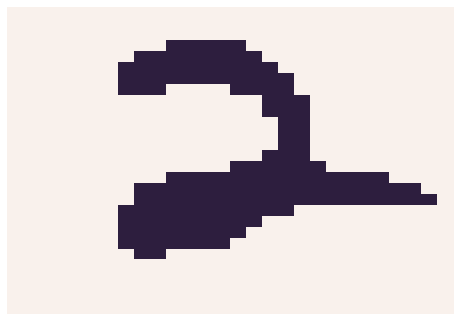Example of a binarized MNIST image. Binarized digits are recognizable, but not so much for natural images.

However, MNIST and its friends are encoded as floating point values in the range [0, 1], where the 256 integers are normalized to lie between these boundaries. There is an expressivity problem here, because Bernoulli variables cannot sample values between 0 and 1!

For papers that train on non-binarized MNIST, we must instead interpret the encoded values as emission probabilities for corresponding Bernoulli variables, i.e. if we see a pixel value of 0.9, it actually represents a Bernoulli likelihood of the pixel being 1, not the sample value itself. The optimization objective is to minimize the cross entropy between predicted probability distribution (parameterized by a scalar emission probability), and the stored emission probability in the data. The cross-entropy of two Bernoullis with emission probabilities $p(1)$ and $p_\theta(1)$ are given by:

$$H(p, p_\theta) = -\left[(1-p(1)) log (1-p_\theta(1)) + p(1) log p_\theta(1)\right]$$

Remember from the earlier section in this post that minimizing this cross entropy results in the same objective as maximizing likelihood! The average log-likelihood (relative entropy) of these toy image datasets is usually reported in units of nats.

The DRAW paper (Gregor et al. 2015) extends this idea to modeling per-channel colors. However, there is a serious drawback to interpreting color pixel data as emission probabilities. When we sample from our generative model, we get noisy, speckly images rather than natural-looking coherent images. Here’s a Python code snippet that reproduces this problem:

 import tensorflow_datasets as tfds import numpy as np import matplotlib.pyplot as plt builder = tfds.builder("cifar10") builder.download_and_prepare() datasets = builder.as_dataset() np_datasets = tfds.as_numpy(datasets) img = next(np_datasets['train'])['image'] sample = np.random.binomial(1,p=img.astype(np.float32)/256) fig, arr = plt.subplots(1, 2) arr.imshow(img) arr.imshow((sample*255).astype(np.uint8))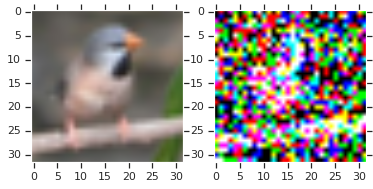Interpreting pixel values as ‘emission probabilities’ results in unrealistic-looking samples - while it is an O.K. assumption for handwritten digits and sprites, it doesn't work for larger-scale, natural images. Papers that do use Bernoulli decoders will often showcase the emission probabilities (e.g. in a reconstruction or imputation task) rather than actual samples.

#### Pixel Values as Categorical Distributions

Larger color datasets (SVHN, CIFAR10, CelebA, ImageNet) are encoded in 8-bit RGB color (each channel is a uint8 integer that ranges in value from 0 to 255, inclusive).

Instead of interpreting their pixel values as Bernoulli emission probabilities, we can attempt to model the distribution over actual uint8 pixel values encoded in the image. One of the simplest choices is a 256-way categorical distribution.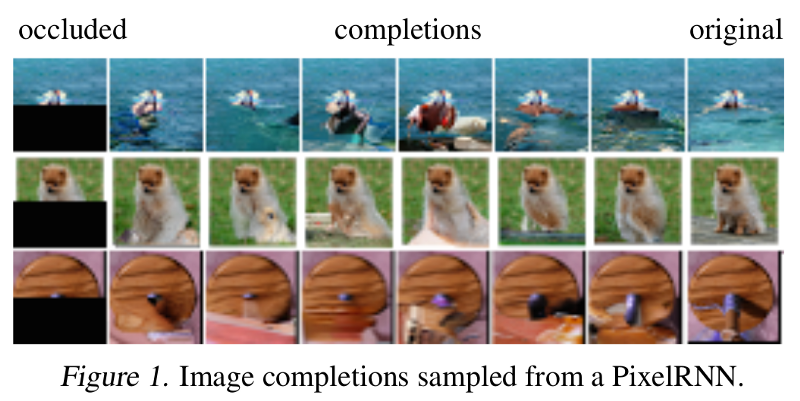Sampling from categorical distributions allows the generative model to sample images rather than emission probabilities.

For color images, it is common to report cross entropies of individual pixels in log base 2, instead of log base e. If a test set with 3072 pixels per image has average likelihood (nats) of $-H(p, q)$, the “bits-per-pixel” is just $-H(p, q)\div log (2)\div3072$.

This metric is motivated by average-likelihood-as-compression interpretation we discussed earlier: for a pixel that is typically encoded using 8 bits, we can devise a lossless entropy coding scheme using our generative model $p_\theta$ that can actually compress the entire dataset with an average bit length of 3 for representing each pixel.

At the time of this writing, the best generative model for Cifar10, Sparse Transformers, achieves a test likelihood of 2.80 bits per pixel. As a point of comparison, PNG and WebP -- widely used algorithms for lossless image compression -- achieve about 5.87 and 4.61 bits on Cifar10 images, respectively (PNG achieves 5.72 bpp if you don’t count the extra bytes like headers and CRC checksums).

This is quite exciting, because it suggests that machine learning can be used for better content-aware entropy-encoding schemes than existing compression schemes. Efficient lossless compression can be used to improve hashing algorithms, make your downloads faster, and improve your Zoom calls, and all of that technology is probably quite feasible today.

#### Stochastic De-Quantization for Continuous Density Models

If we optimize a continuous density model (such as a mixture of Gaussians) to maximize log-likelihood on discrete data, this can result in a degenerate solution where the model assigns the same density spike to each of the possible discrete values {0, ..., 255}. Even with an infinitely large dataset, the model can achieve arbitrarily high likelihoods by simply squeezing the spikes narrower and narrower.

To address this problem. it is quite common to de-quantize the data by adding noise to the integer pixel values. One such transformation is given by $y = x + u$, where $u$ is a sample from the random uniform $U(0,1)$. The first paper that I am aware of that motivates stochastic de-quantization for density modeling is Uria et al. 2013, and has since become common practice in Dinh et al., 2014, Salimans et al., 2017, and the works that built on top of these papers.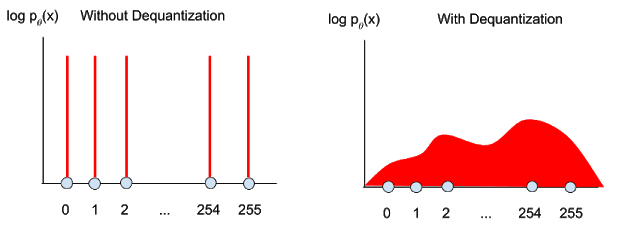Left: optimizing density models on discrete data can result in a degenerate solution where the model assigns a probability spike on a finite set of discrete values. Stochastic de-quantization is often applied so that we learn likelihood models on continuous data.

A discrete model assigns probability mass over an interval, while a continuous model assigns a density function. Let $P(x)$ and $p(x)$ represent the discrete probability masses and continuous densities of the true data distribution, and let $P_\theta(x)$ and $p_\theta(x)$ represent the same for the model density. We’ll derive below why optimizing the continuous likelihood model $p_\theta(y)$ over de-quantized data $y$ results in optimizing the lower-bound of the actual discrete probability model $P_\theta(x)$:

Integrating the density over a unit interval gives us the total mass implied by the density function:

$$P_\theta(x) = \int_0^1 p_\theta(x+u) du$$

Our model likelihood objective is trained on de-quantized data sampled from the true data distribution:

$$\mathbb{E}_{y \sim p}\left[ \log p_\theta(y) \right]$$

By definition of expectation:

$$= \int p(y) \log p_\theta(y) dy$$

Expanding the integral,

$$= \int dy p(y) \int dy \log p_\theta(x+du)$$
$$= \mathbb{E}_{x \sim P} \int du \log p_\theta(x+du)$$

Via Jensen’s inequality (for the uniform variable u),

$$\leq \mathbb{E}_{x \sim P} \log \int du p_\theta(x+du)$$
$$= \mathbb{E}_{x \sim P} \log P_\theta(x)$$

A recent paper, Flow++, proposes using a learned de-quantization random variable to improve the tightness of the variational bound. The intuition here is that a single importance-sampled noise variate from $q(u|x)$ results in a lower-variance estimate of the integral $\int_0^1 p_\theta(x+u) du$ than a single sample from a uniform(0, 1) distribution. Because the de-quantization noise is different, one consequence of this work is that density models with different architectures and different quantization strategies cannot be compared in a controlled manner.

One way to compare Flow++ and uniformly de-quantized generative models fairly is to permit researchers to use whatever variational bound they like at training time, but standardize the evaluation of likelihood at evaluation time to be some tight multi-sample bound. The intuition here is that as we integrate over more samples, we get a better approximation of the true log-likelihood of the corresponding discrete model $P_\theta(x)$.

For instance, we could report the multi-sample bound from a fixed U(0, 1) de-quantization distribution, as commonly done in VAE literature with multi-sample IWAE bounds. A discussion of VAEs and IWAE bounds are out of the scope of this tutorial, and will be covered in the future.

#### Side Note: Data Preprocessing for Normalizing Flows

Normalizing Flows are a family of generative models that “transform” a base probability distribution into a more complicated probability distribution.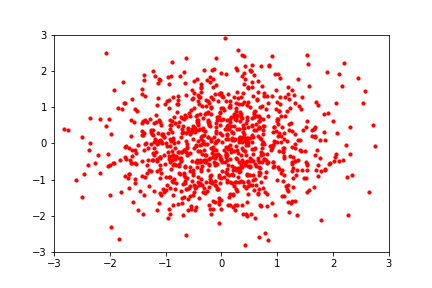Normalizing Flows learn transformations that have tractable inverses and Jacobian determinants. Being able to compute these two quantities efficiently allow us to calculate the transformed distribution’s log-density, using the change-in-variables rule:

$$\log p(y) = \log p(x) - \log |det J(f)(x)|$$

The vast majority of Normalizing Flows operate over continuous density functions (thus requiring the volume-tracking Jacobian determinant term), though there is some recent research on “discrete flows” that learn to transform probability mass functions rather than transforming density (Tran et al. 2019, Hoogeboom et al. 2019). We won’t be discussing these flows much in this blog post, but suffice it to say that they work by devising bijective discrete transformations of discrete base distributions.

In addition to the stochastic de-quantization mentioned earlier, there are a couple additional tricks employed when training normalizing flows for image data.

Empirically, re-scaling the data from the range [0, 256] to the unit interval [0, 1] prior to maximum likelihood estimation helps stabilize training, as neural network biases are usually centered around zero.

To prevent boundary issues where a sample from the base distribution could get mapped to a point outside of the re-scaled boundary (0, 1) by the flow, we can transform the re-scaled data to the range $-\infty, \infty$ via the logistic function (the inverse of the sigmoid function).

We can think of these re-scaling and logistic transforms as "preprocessing" flows at the beginning of the model, where just like any other bijector, we have to account for the change in volume induced by the transformation.

The important thing to realize here is that for evaluation purposes, pixel densities should always be computed in the continuous interval [0, 256], so that we can compare likelihoods from flows and autoregressive over the same data (up to the variational gap induced by the stochastic dequantization).

Here is a diagram showing a standard normalizing flow for RGB images, with the original discrete data on the left and the base density (can be a Gaussian, a logistic, or whatever your favorite tractable density is) on the right.Generative model likelihoods typically reported in de-quantized space (green). Starting from Dinh et al. 2016, many flow-based models re-scale pixels to $[\lambda, 1-\lambda]$ and apply the logistic function (inverse sigmoid) to help with numerical stability on boundary conditions.

#### Discretized Logistic Mixture Likelihood

One drawback of modeling pixels with categorical distributions is that a categorical cross entropy loss cannot tell us that a pixel value of 127 is closer to 128 than it is to 0. For an observed pixel value $p$, the gradient of the categorical cross entropy is constant with respect to pixel intensity (since the loss treats the categories as un-ordered). Although the cross entropy gradient is non-zero, it is said to be “sparse” because it does not provide information on how close (in pixel intensity) we are to the target distribution. Ideally, we would like gradients to be larger in magnitude when the predicted intensity is far away from the observed value, and smaller when the model is close.

A more serious problem with modeling pixels as categorical distributions is that if we choose to represent more than 256 categories, we’d be in trouble. For example, we might want to model the R, G, and B pixels jointly (256^3 categories!) or model higher-precision pixel encodings than uint8 for HDR images. We’d quickly run out of memory attempting to store the projection matrices needed to map neural net activations to logits for that many categories.

Two concurrent papers, Inverse Autoregressive Flow and PixelCNN++, solve these problems by introducing a probability distribution for modeling RGB pixels as ordinal data, for which gradient information from the cross entropy loss can push pixels in the right direction while still preserving a discrete probability model.

We can model continuous pixel probability densities via a mixture of logistics, which is a continuous distribution. To recover probability mass for discrete pixel values, we can use the convenient property of the logistic distribution is that its CDF is the sigmoid function. By subtracting two sigmoids CDF(x+0.5) - CDF(x-0.5), we can recover the total probability mass lying between two integer pixel values.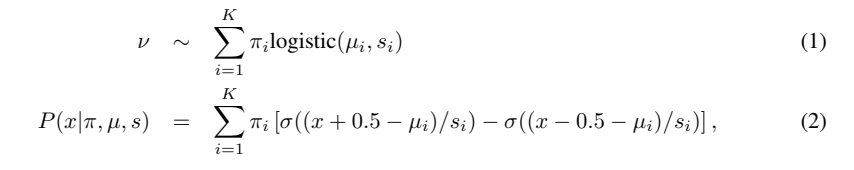For example, the probability of a pixel having value=127 is modeled as the probability mass lying between 126.5 and 127.5 for a continuous mixture of logistic distributions. The probability model must also account for edge cases such that CDF(0-0.5) is 0 and CDF(255+0.5) is 1, as is required of probability distributions.

Representing pixels in this way also affords the luxury of being able to handle a lot more categories, which means that PixelCNN++ can model the R, G, and B pixel channels at once. The caveat here is that you must tune the number of mixture components adequately (on Cifar-10, 5 seems to be enough).

Analogous to how Tran et al. 2019 devise discrete flows on top of categorical distributions, Hoogeboom et al. 2019 devise discrete flows for ordinal data by using this discretized logistic mixture likelihood as the base distribution. This gets the best of both worlds: we get to use normalizing flows for tractable inverses and sampling, while avoiding to have to solve for a de-quantized likelihood objective (which may incur a variational lower bound penalty on the likelihood). Both are very exciting papers that I hope to write about more in the future!

### Perplexity

Log-likelihood is also a common metric for evaluating generative models in the language modeling domain. A discrete alphabet without ordering makes Categorical distributions the most natural choice for density modeling.

One quirk of the natural language processing (NLP) field is that language model likelihoods are often evaluated in units of Perplexity, which is given by $2^H(p, q)$. The inverse of perplexity, $\log_2 2^-H(p, q)$, is nothing more than average log-likelihood $-H(p, q)$. Perplexity is an intuitive concept since inverse probability is just the "branching factor" of a random variable, or the weighted average number of choices a random variable has. The relationship between perplexity and log-likelihood is so straightforward that some papers (Image Transformer) actually use the word “perplexity” interchangeably with log-likelihoods.

### Closing Thoughts

In this blog post we derived the relationship between maximizing average log-likelihood and compression. We also mentioned several modeling choices between discrete and continuous likelihood models for individual pixels.

There is a broader question of whether likelihood is even the right quantity to be measuring / optimizing. At NIPS 2016 (now known as the NeurIPS conference), I recall there being a pretty lively debate in the generative modeling workshop where people were debating whether optimizing tractable-likelihood models was even a good idea.

It turns out that optimizing and evaluating against likelihood was a good idea after all, because since then researchers have figured out how to build and scale up much more flexible likelihood models while keeping them computationally tractable. Models like Glow, GPT-2, WaveNet, and Image Transformer are trained with likelihood and can generate image, audio and text samples with stunning quality. On the other hand, one might argue that at the end of the day, generative modeling needs to be coupled to performance on an actual task, such as classification accuracy when the model is fine-tuned on a labeled dataset. My colleague Niki Parmar says the following about images vs text likelihood models:
On text, there is generally a pattern where better likelihood leads to better performance on downstream tasks like GLUE. On images, I've heard from other practitioners that pixel prediction doesn't work as a pre-training task for downstream tasks like image classification. It could be because pixels don't mean much in terms of representations as compared to word-pieces or words in text. It's an open question but I find it fascinating that representation learning in images is quite different, almost difficult to establish and measure.
In a future blog post, I’ll build on top of this tutorial and discuss the evaluation of generative models that optimize variational lower bounds on log-likelihood (e.g. Variational Autoencoders, importance-weighted autoencoders).

• Twitter Thread discussing alternative metrics to the commonly reported log-likelihood and IWAE bounds.
• If you’re curious about what the compression ratios are for common lossless image compression algorithms, check out this script and paper.
• See the PixelRNN paper and this Reddit thread for further discussion on why categorical classification is preferable to continuous density modeling.
• Proper Local Scoring Rules - Thanks to Ferenc Huszár for pointing this paper out to me.
• A Note on the Evaluation of Generative Models - A terrific paper by Theis et al. that is a must-read for anyone getting started in the field of generative modeling.
• On the Quantitative Analysis of Decoder-based Generative Models
• Tutorial and Derivation of Perplexity, and this Stack Exchange question on Perplexity. Being unfamiliar with NLP myself, these links were super helpful for learning about the topic.
• The generative modeling community is fairly consistent about reporting MNIST in nats and color images in bits-per-dim, though some papers report MNIST likelihoods in bits/dim and others will report CIFAR10 in nats.
• This paper by Ranganath et al. proposes a general framework for thinking about variational inference (e.g. VAEs) by moving away from the standard KL divergence objective. One first imagines the desiderata they’d like to see in their divergence measure (e.g. sampling, preventing mode collapse), and then the paper proposes a method to recover a variational objective for the desired divergence. Thanks to Dustin Tran for pointing this one out to me.

### Acknowledgements

Many thanks to Dustin Tran, Niki Parmar, and Vincent Vanhoucke for reviewing drafts of this blog post. As always, thank you for reading!